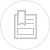# various other units

#### becquerel (Bq)

This is the unit of measurement applicable to radioactivity. The activity of a radioactive source is equal to the number of disintegrations per unit of time. The Becquerel refers to a disintegration (or transformation of an atom) per second.

#### gray (Gy)

Radiation transmits its energy to the matter they passe through. The dose absorbed is the average energy absorbed per unit of mass of matter and is expressed in grays :

#### sievert (Sv)

For the same dose, the effect produced by various radiations on a living organism changes according to the nature of the organism and to the organs exposed.

With regard to radioprotection, we first have to establish the RBE (relative biological effectiveness) of the radiation: this effectiveness is equal to the ratio, between doses of a reference radiation and the radiation under examination, that produce the same biological effect, and this effect increases as the dose reduces.

The equivalent dose is the product of the dose absorbed by the RBE; it is expressed in sievert (Sv).

When stochastic effects and low doses are involved, this coefficient is termed the quality factor (QF) :

Note: the current preference consists in introducing two successive concepts: equivalent dose, multiplying the absorbed dose by a weighting factor linked to the nature of the radiation + effective dose, multiplying the equivalent dose by a weighting factor linked to the nature of the tissues.

These doses are calculated; the unit used is again the sievert (or J · kg–1).

The old radioactivity units, the Curie, Rad and Rem, although unofficial, are still used in some countries.

## unit of colour

The colour of a water sample is measured by comparing it visually with a series of master samples containing known quantities of potassium chloroplatinate and cobalt chloride (II).

A unit of true colour (UTC), or a platinum-cobalt unit, refers to the quantity of colour revealed under specified sampling conditions, by a control solution containing 1 mg of platinum per litre.

## turbidity unit

Turbidity is the optical property of water such that the incident light is diffused and absorbed instead of being transmitted in a straight line through a sample.

Nephelometric turbidity metres measure the intensity of the light diffused at an angle of 90° in relation to the incident light. The apparatus’ readout unit is expressed in nephelometric formazine units (NFU).

Formazine suspensions are used as the main control reference. A formazine suspension produced by the reaction between hydrazine sulphate (50 mg·L–1) and hexamethylene-tetramine (500 mg·L–1) under stringent conditions has a turbidity of 40 NTU.

## Bookmark tool